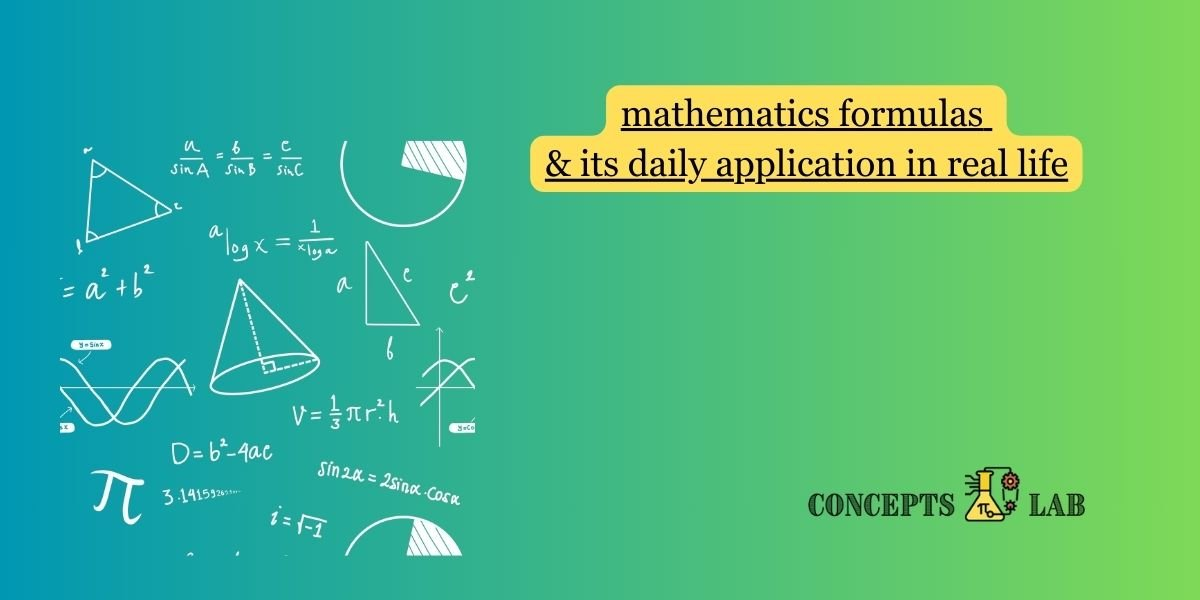Mathematics plays a crucial role in various aspects of daily life, from simple calculations to complex problem-solving. Here are some common mathematical formulas and their daily applications:

1. Area of a Rectangle (A = l * w):
• Application: Calculating the area of a room or a piece of land.
2. Volume of a Cylinder (V = π * r^2 * h):
• Application: Determining the amount of liquid a cylindrical container can hold, like a water tank or a soda can.
3. Simple Interest (I = P * R * T / 100):
• Application: Calculating interest on loans, savings, or investments.
4. Pythagorean Theorem (a^2 + b^2 = c^2):
• Application: Measuring distances, such as when constructing buildings or determining the length of a ladder needed to reach a certain height.
5. Quadratic Formula (x = \frac{-b \pm \sqrt{b^2-4ac}}{2a}x=2ab±b2−4ac​​):
• Application: Solving quadratic equations, which can arise in various real-world problems.
6. Percentage Change (\frac{New – Old}{Old} \times 100%OldNewOld​×100):
• Application: Calculating changes in prices, discounts, or increases/decreases in values.
7. Gross Domestic Product (GDP) Calculation:
• Application: Assessing the economic health of a country.
8. Probability (P(A) = \frac{\text{Number of favorable outcomes}}{\text{Total number of outcomes}}Total number of outcomesNumber of favorable outcomes​):
• Application: Predicting the likelihood of events, such as weather forecasts or game outcomes.
9. Compound Interest (A = P (1 + \frac{r}{n})^{nt}(1+nr​)nt):
• Application: Computing interest on savings or investments that compound over time.
10. Speed, Distance, Time Relationship (Speed = \frac{\text{Distance}}{\text{Time}}TimeDistance​):
• Application: Planning travel time or calculating the speed of a moving object.
11. Binomial Distribution Probability (P(X = k) = \binom{n}{k} p^k (1-p)^{n-k}P(X=k)=(kn​)pk(1−p)nk):
• Application: Used in statistics to model the number of successes in a fixed number of independent trials.
12. Linear Regression Equation (y = mx + b):
• Application: Predicting trends and relationships between variables, commonly used in data analysis.
13. Newton’s Law of Universal Gravitation (F = \frac{G \cdot m_1 \cdot m_2}{r^2}r2Gm1​⋅m2​​):
• Application: Calculating gravitational forces between objects, crucial in physics and astronomy.
14. Euler’s Formula (e^{ix} = \cos(x) + i \sin(x)eix=cos(x)+isin(x)):
• Application: Used in complex number analysis, signal processing, and electrical engineering.

These are just a few examples, and mathematics is deeply woven into everyday life in various fields, including finance, engineering, science, and technology. Understanding and applying mathematical concepts can enhance problem-solving skills and contribute to informed decision-making.